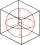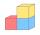# Cube + expression of a variable from the formula - math problems

#### Number of problems found: 88

• Cube 5The content area of one cube wall is 32 square centimeters. Determine the length of its edges, its surface and volume.
• Body diagonalCalculate the volume and surface of the cube if the diagonal body measures ten dm.
• CalculateCalculate the surface of the cube if its volume is 64 cm ^ 3.
• Cube diagonalsCalculate the length of the side and the diagonals of the cube with a volume of 27 cm3.
• Inscribed circleA circle is inscribed at the bottom wall of the cube with an edge (a = 1). What is the radius of the spherical surface that contains this circle and one of the vertex of the top cube base?
• CalculateCalculate the length of the wall diagonal of the cube with an edge 5 cm long.
• Sum of the edgesThe sum of the lengths of all edges of the cube is 72 cm. How many cm2 of colored paper are we going to use for sticking?
• Cube 7Calculate the volume of a cube, whose sum of the lengths of all edges is 276 cm.

We apologize, but in this category are not a lot of examples.
Do you have an exciting math question or word problem that you can't solve? Ask a question or post a math problem, and we can try to solve it.

We will send a solution to your e-mail address. Solved examples are also published here. Please enter the e-mail correctly and check whether you don't have a full mailbox.

Cube Problems. Expression of a variable from the formula - math problems.